# Removing Blanks

There are a number of different Array Formulas that you can use to achieve this.
Each one has its own advantages and disadvantages.
You don't have to use Array Formulas, there are other ways of doing this.
Cells & Ranges > Removing Blank Cells Horizontal
Cells & Ranges > Removing Blank Cells Vertical
Remember all the formulas on this page must be entered with (Ctrl + Shift + Enter) if you are not using Excel 365.
In Excel 365, array formulas are native and do not require Ctrl + Shift + Enter.

### Example 1 - Vertical

`=IF(ROWS(D\$2:D2)>COUNTA(B:B),"",INDEX(B:B,SMALL(IF(B\$2:B\$12<>"",ROW(B\$2:B\$12)),ROWS(D\$2:D2)))) `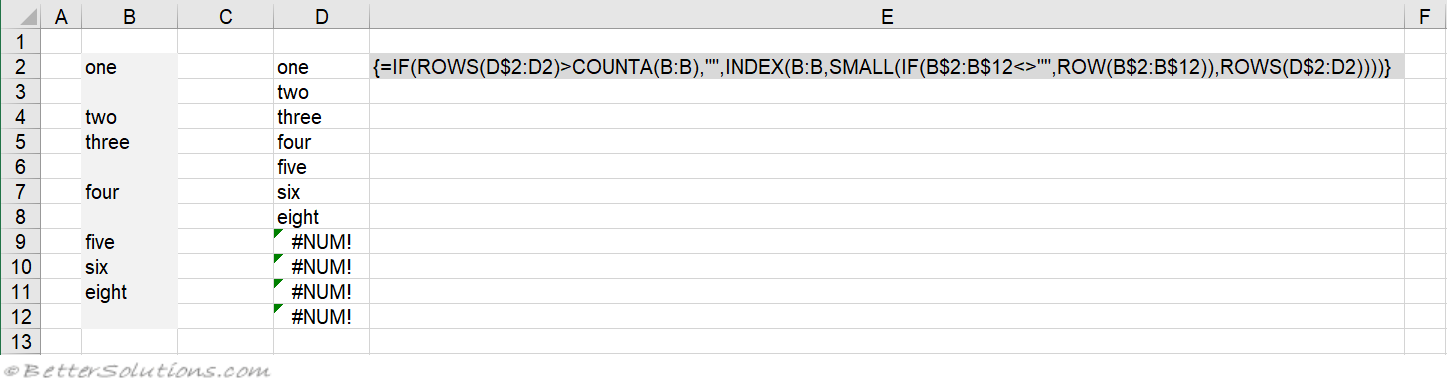assumes that there is nothing else in this column
can be dragged down
doesn't need to be on the same rows
only works on vertical lists

### Example 2 - Vertical

`=IF(COUNTIF(\$B\$2:\$B\$12,"?*")<ROW(B2)-1,"",INDEX(B:B,SMALL(IF(B\$2:B\$12<>"", ROW(B\$2:B\$12)),ROWS(B\$2:B2)))) `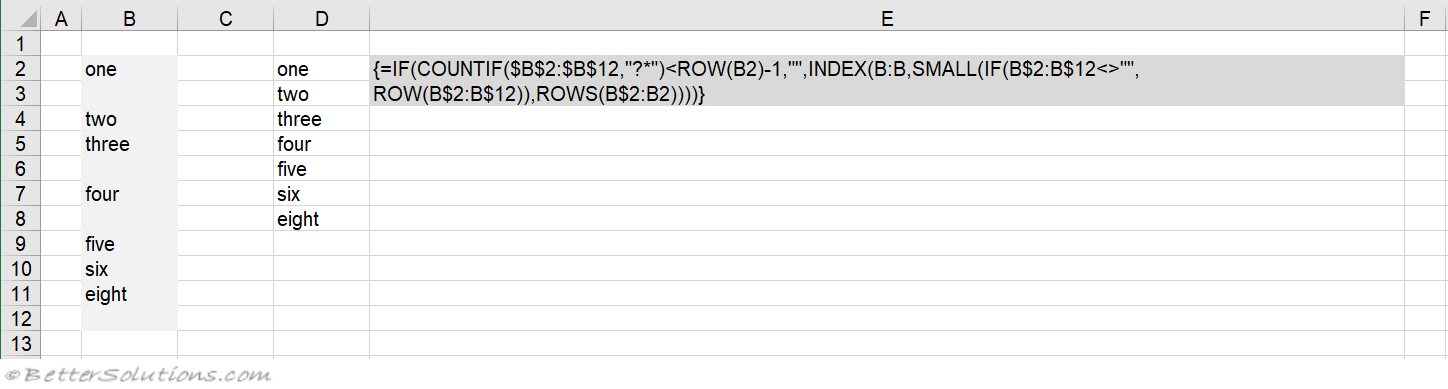can be used with other data in the column
can be dragged down
doesn't need to be on the same rows
only works on vertical lists

### Example 3 - Vertical

`=IF(ISERROR(SMALL(IF(B2:B12<>"",ROW(B2:B12)-1),ROW(B2:B12)-1)),"", INDEX(B2:B12,MATCH(SMALL(IF(B2:B12<>"",ROW(B2:B12)-1),ROW(B2:B12)-1), IF(B2:B12<>"",ROW(B2:B12)-1),0))) `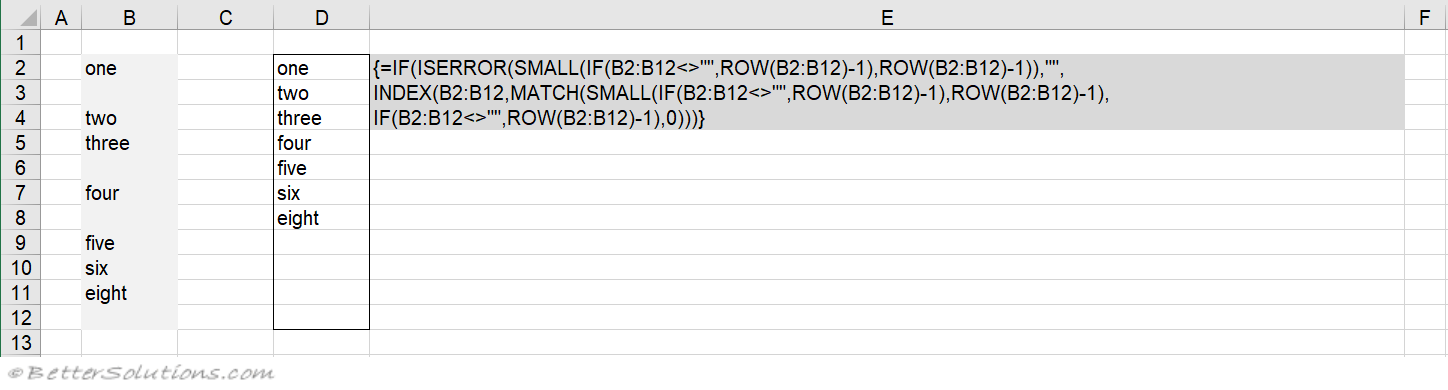must be entered as one array formula block and cannot be dragged down
must enter the row number to subtract from the current ROW (in this example 1)
doesn't need to be on the same rows
only works on vertical lists

### Example 4 - Vertical

Thank you to Chip Pearson (cpearson.com/excel/NoBlanks.aspx)

`=IF(ROW()-ROW(\$B\$2:\$B\$12)+1>ROWS(\$B\$2:\$B\$12)-COUNTBLANK(\$B\$2:\$B\$12),"", INDIRECT(ADDRESS(SMALL((IF(\$B\$2:\$B\$12<>"",ROW(\$B\$2:\$B\$12),ROW()+ROWS(\$B\$2:\$B\$12))), ROW()-ROW(\$D\$2:\$D\$12)+1),COLUMN(\$B\$2:\$B\$12),4))) `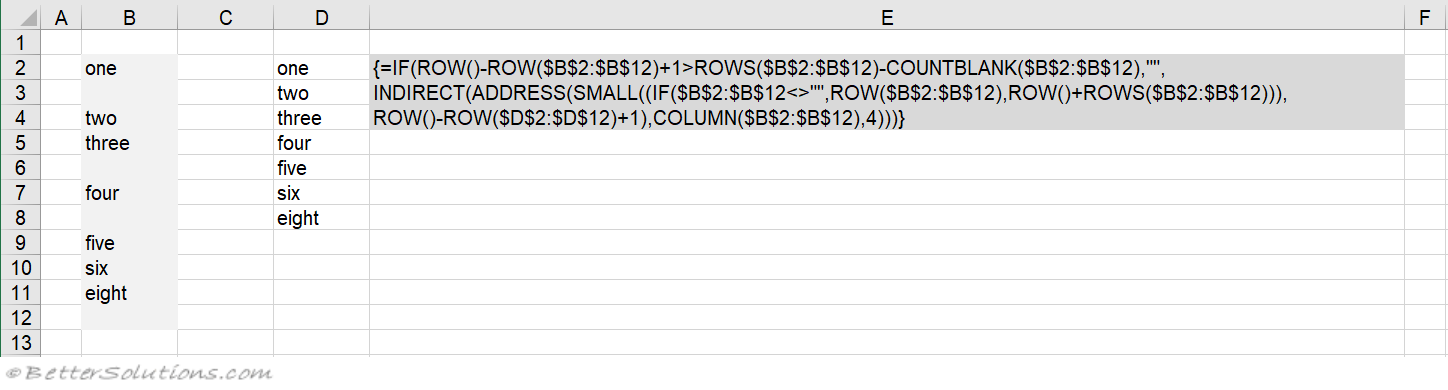can be dragged down
doesn't have to be on the same rows
only works on vertical lists

### Example 5 - Horizontal

`=IF(COLUMN(B:B)<=SUM(--(\$B\$2:\$L\$2<>""))+1,INDEX(\$B\$2:\$L\$2,0,SMALL(IF(\$B\$2:\$L\$2<>"", COLUMN(\$B\$2:\$L\$2)-1,""),COLUMN(B:B)-1)),"") `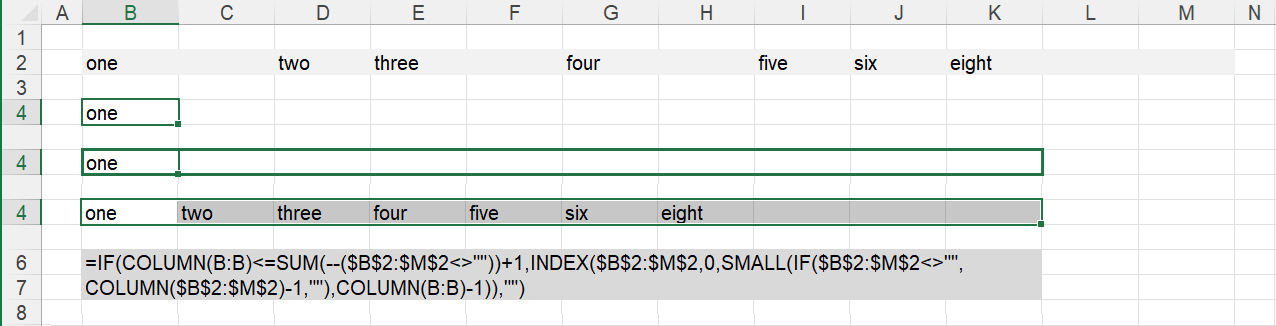doesn't need to be in the same columns
can be dragged across
only works on horizontal lists

© 2022 Better Solutions Limited